﻿ Analyzing the Stock Market Using the Solution of the Fractional Option Pricing ModelPublications are Open
Access in this journal
Article Versions
Export Article
• Normal Style
• MLA Style
• APA Style
• Chicago Style
Research Article
Open Access Peer-reviewed

### Analyzing the Stock Market Using the Solution of the Fractional Option Pricing Model

Osu B. O , Chukwunezu A. I, Olunkwa C., Obi C. N
International Journal of Partial Differential Equations and Applications. 2019, 6(1), 1-12. DOI: 10.12691/ijpdea-6-1-1
Received February 17, 2019; Revised April 21, 2019; Accepted May 02, 2019

### Abstract

The aim of this work is to analyze the stock market using the solution of the fractional option pricing model as in literature. First, the Hurst exponent of the stock prices of two different stock index using Detrended Fluctuation Analysis (DFA) method was estimated. A program using MATLAB code was written which is used to calculate the Hurst exponent, the volatility, the discount rate, the call and put options prices efficiently so as to save time and avoid computational errors which may arise through manual computation.

### 1. Introduction

The Black-Scholes model was first developed by Fisher Black and Myron Scholes in 1973. They derived a partial differential equation, now called the Black-Scholes equation, which estimates the price of the option over time. The key idea behind the model is to hedge the option by buying and selling the underlying asset in just the right way and as a consequence, to eliminate risk. Merton 1 was first to publish a paper expanding the mathematical understanding of the options pricing model and coined the term “Black-Scholes option pricing model. Black-Scholes model does not give the value of the option (the price at which it should be sold or bought) directly. In order to determine the value of an economic asset such as stock, one needs to take into account at least two aspects of random variability: the growth of the asset and the stability of stock market price. Osu et al. 2 considered a stability analysis of stochastic model of price change at the floor of a stock market. They determined the equilibrium price and growth rate of the stock shares in a particular case by establishing a dynamic stochastic model under certain conditions.

Carr and Madan 3 introduced fast Fourier transform algorithm for the numerical value of the Fourier integral encountered in option pricing. Since then, fast Fourier transform algorithm has become an efficient mathematical tool used in mathematical finance in the valuation problems. A more general pricing framework was developed in Lewis 4 by separating the underlying asset price from the option payoff through the use of Plancheral-Parseval theorem. Dennis 5 showed explicitly how to obtain the solution of the classical Black-Scholes equation for call option pricing using the Green’s function for the diffusion equation. Coppex 6 used change of variables to transform the classical Black- Scholes equation into a heat equation. He then applied the heat equation formula to obtain the solution of the Black-Scholes equation.

The arbitrage possibility in the fractional Black-Scholes model depends on the definition of the stochastic integral. More precisely, if one uses the Wick-Ito-Skorohod integral, one obtains an arbitrage-free model. On the other hand, it is easy to give arbitrage examples in continuous time trading with self-financing strategies, if one uses the Riemann-Stieltjes integral. Tommi and Esko 9 discussed the connection between two different notions of self-financing portfolios in the fractional Black-Scholes model by applying the known connection between these two integrals.

Application of Sturm-Liouville Equation to the Solution of the Black –Scholes equation has been presented in Osu et al. 7. An analytical solution of a non-linear Black- Scholes- equation (Option Pricing Model) with transaction cost measure and volatile portfolio risk measure has been presented. The analytical solution was obtained by using variational iteration method in Osu et al. 7.

The aim of this study however is to solve Black-Scholes option pricing equation modeled by Fractional Brownian motion with specified boundary conditions. The equation is solved using change of independent variables and Fourier transform.

### 2. The Model

Given the equation as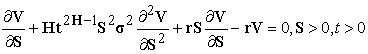(2.1)

withas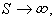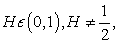and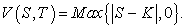Where equation (2.1) is called Black-Scholes option pricing equation modeled by Fractional Brownian motion. Equation (2.1) can be reduced to a one-dimensional heat equation of the form 8;(2.2)

Equation (2.2) is a one-dimensional heat equation, which have been solved using Fourier transform with solution given as(2.3)

THEOREM 2.2: Let the solution of the heat equation be given as in (2,3), then the solution of (2,1) can be obtained from (2,3) in the form(2.4)

wherePROOF: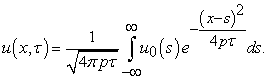We need to transform the initial condition too. From (4.14b), we have: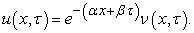But from (2.5b), we have that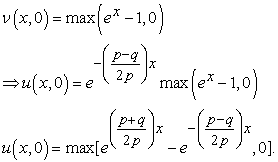(2.5a)

We will make a change of variable in the integration in (2.4) by takingThus, the integration becomes: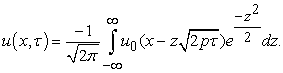We will only integrate over the domain where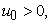that is for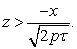On that domain,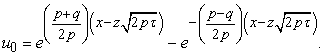So, we have: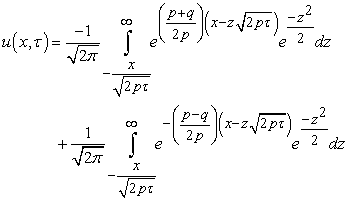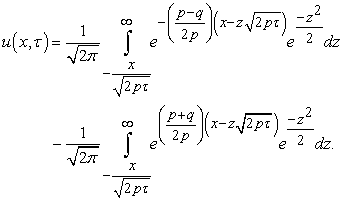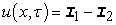(2.5b)

where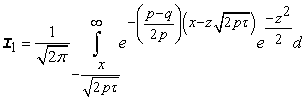(2.5c)(2.5d)

Evaluation of: To evaluate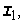consider the exponent;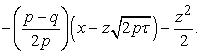Expanding we have: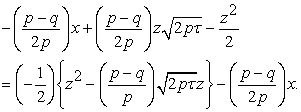Completing the square, we have: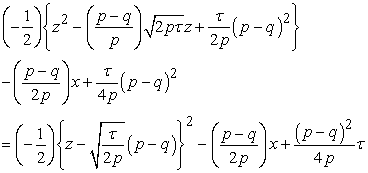Thus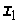becomes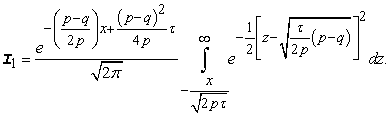We will change variables again on the integral. Choose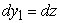This integral can be represented in terms of the cumulative distribution function of a normal random variable, usually denoted byThat is:see DunbarThus,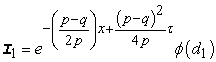whereEvaluation of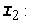To evaluate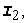consider also the exponent;Expanding we have: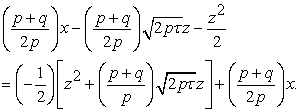Completing the square, we have:Thusbecomes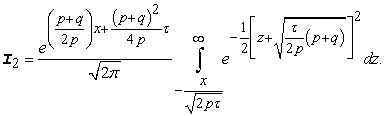We will change variables again on the integral. ChooseThis integral can be represented in terms of the cumulative distribution function of a normal random variable, usually denoted byThat is:Thus,(2.7)

whereThus (2.5b) becomes:(2.8)

We will unwind each of the changes of variables:

Recall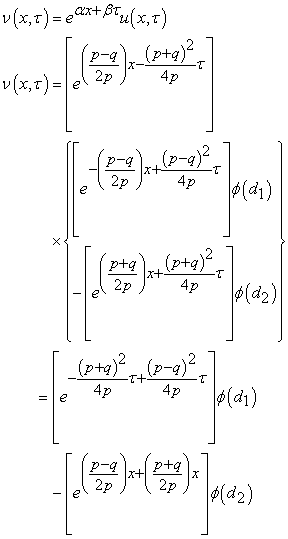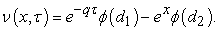But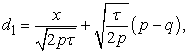(2.9)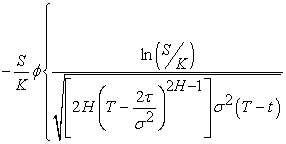But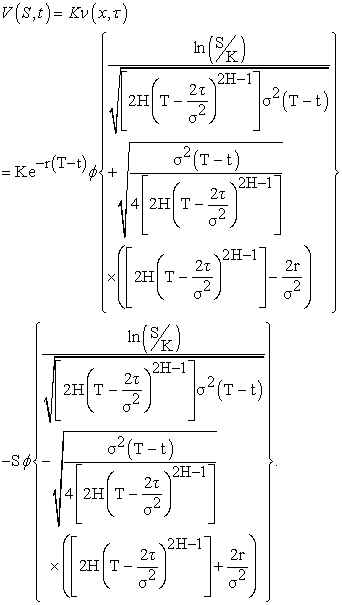So that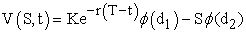(2.10)

whereHence the proof.

Note that the values ofand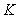can be obtained from daily stock market prices; see Table 1 and Table 2. The values of other parameters can be obtained using the methods given in chapter three.

Equation (2.10) is the solution of the Black-Scholes call option pricing equation modeled by fractional Brownian motion. It is the formula for obtaining the call option price. We will use the put-call parity equation to find the formula for obtaining the corresponding put option price. The put-call parity equation is stated inas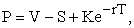(2.11)

where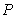is the put option price;is the call option price;is the strike price;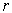is the discount rate and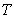is the expiration time.

Substitutinginwe have(2.12)

### 3. Application to the Stock Market

To illustrate how option prices can be calculated, we made use of daily stock price lists of Conoil and Cutix stock index which were obtained online from Cash Craft Asset Management Limited from January 2016 to December 2016. A total of 230 stock prices were obtained for Conoil while a total of 200 stock prices were obtained for Cutix.

• Figure 1. Graph of LogF(n) against logn for Conoil. The slope of the graph is 0.86, thus the Hurst exponent is 0.86
• Figure 2. Graph of LogF(n) against logn for Cutix. The slope of the graph is 0.47, thus the Hurst exponent is 0.47

3.2.1. The Graphical Representation of the Call and Put Option Prices

The results of the call and put option prices for Conoil and Cutix stock index are represented in the following graphs:

• Figure 3. Graph of call option prices for conoil against the stock prices, s and the time to maturity, t with varying strike prices,k and varying discount rate,r.(chosen parameters: Hurst exponent, H=0.86, volatility, σ=4.346, length of the period in years, τ=0.003)

From the graph, we notice that the call option values move downward and upward in similar pattern, thus it will be possible to predict the pattern the subsequent call option values will take.

• Figure 4. graph of put option prices for conoil against the stock prices, S and the time to maturity, t with varying strike prices, k and varying discount rate,r. (chosen parameters: Hurst exponent, H=0.86, volatility,σ=4.346, length of the period in YEARS, τ=0.003)

From the Figure 4 above, we notice that the put option values move downward and upward in similar pattern too, thus it will be possible to predict the pattern subsequent put option values will take.

From the graph of Figure 5, we notice that the movement of the call option values is completely random, thus it will be difficult to make predictions on subsequent call option values

• Figure 5. Graph of call option prices for cutix against the stock prices, S and the time to maturity, t WITH varying strike prices, K and varying discount rate,r. (Chosen parameters: Hurst exponent, H=0.47, volatility, σ=0.488, length of the period in years, τ=0.003.
• Figure 6. Graph of put option prices for Cutix against the stock prices, S and the time to maturity,t with varying strike prices,K and varying discount rate,r. (Chosen parameters: Hurst exponent, H=0.47, volatility, σ=0.488, length of the period in years, τ=0.003)

Figure 6 shows that the movement of the put option values is completely random as that of the call option, thus it will be difficult to make predictions on subsequent put option values.

### 4. Conclusion

In this work, we have used the formula we have obtained by solving the Black-Scholes option pricing equation modeled by fractional Brownian motion using change of variables and Fourier transform to analyze and study option pricing. Increase in volatility increases option value (both call and put options) while decrease in volatility decreases option values. Furthermore, increase in the value of the Hurst exponent also increases the option values, while decrease in the value of the Hurst exponent also decreases the option values. We discovered too that the higher the value of the Hurst exponent, the easier it is to predict option values. Prediction of option values can be very difficult when the value of the Hurst exponent is very small

### Acknowledgements

Manifold thanks to the reviewers and those cited herein.

### References

  Merton, R. (1997). Theory of rational option pricing, Bell Journal of Economics and management science, 4(1), 141-183. In article View Article  Osu B. O., Okoroafor, A. C. and Olunkwa, C. (2009); Stability analysis of stochastic model of stock market price, African Journal of Mathematics and Computer science Research, 2(6), 98-103. In article  Carr, P.P. and Madan, D.B. (1999). Option valuation using the Fast Fourier transform, Journal of computational finance 2(4), 61-73. In article View Article  Lewis, A. (2001). A simple option formula for general Jump-Diffusion and other Exponential Levy processes, Envision financial systems and option city.net, California, available at http/optioncity.net/pubs/explevypdf. In article View Article  Dennis, S. (1999). Solution of the Black-Scholes equation using the Green’s function of the Diffusion Equation, Department of Physics and Astronomy, 4129 Frederick Reines. Hall, University of California Irvine, Irvine, CA 92697-4575. In article  Coppex, F. (2009). Solving the Black-Scholes Equation: a demystification. URL: http://WWW.francoiscoppex.com. In article  Osu, B. O., Olunkwa, C., Akpanta, A.C. and Onwuegbula, C. (2016). An Application of Sturm-Liouville Equation to the Solution of the Black-Scholes equation with Transaction cost and Portfolio Risk Measures, Transactions of NAMP, 2, 307-312. In article  Chukwunezu, A. I., Osu, B. O., Olunkwa, C. and Obi, C. N. (2019). On the solution of fractional option pricing model by convolution theorem. Earthline Journal of Mathematical Sciences, 2(1): 143-157. In article View Article  Tommi, S. and Esko, V. (2003); On Arbitrage and Replication in the fractional Black-Scholes pricing model, Statistics and Decisions, Oldenbourg Verlag, Munchen, 21, 137-151. In article

### Appendix A

MATLAB PROGRAM ON HOW TO CALCULATE HURST EXPONENT, VOLATILITY,CALL AND PUT OPTION PRICES

%% This part reads the files for stock and strike and assigns them to S and K respectively

data = xlsread('StockStrikeConoil.xlsx'); % To compute for Cutix, simply replace StockStrikeConoil.xlsx with StockStrikeCutix.xlsx

%% This part computes X,Y,Z,F(n),log(n) and log(F(n))

xx = (1:length(data(:,2)))';

n= 20:20:100;% values of n to be used

logF=[];logn=[];F=[];% empty matrix to store logF,logn and F(n)

for rr = 1:length(n)

numberofy = length(xx)-rem(length(xx),n(rr));

U = [];

for i = 1:length(data(1:numberofy,2))

U(i,1) = data(i,2) - mean(data(1:numberofy,2));

end

nn=numberofy/n(rr);

y=data(1:numberofy,2);

x=[];xxx=(1:n(rr))';

b=[];a=[];

for k=1:nn

x = [(1:n(rr))';x];

yyy = y(k*n(rr)-n(rr)+1:k*n(rr));

b(1,k) = ((n(rr)*sum(xxx.*yyy))-(sum(xxx)*sum(yyy)))/(n(rr)*sum(xxx.^2)-(sum(xxx))^2);

a(1,k) = mean(yyy)- b(1,k)*mean(xxx);

end

z=[];ll=0;

for j=1:numberofy

if rem(j,n(rr))==1

ll=ll+1;

end

z(j,1)=a(1,ll)*x(j)+b(1,ll);

end

f(1,rr) = sqrt((1/numberofy)*sum((U(1:numberofy,1)-z).^2));% PROBLEM HERE

logn(1,rr) = log10(n(1,rr));

logf(1,rr) = log10(f(1,rr));

end

fprintf('%9 s %15 s %21 s \n \n','n','Log (n)','log (F(n))')% prints the tittles n,logn and log(F(n))

fprintf('%9 .3f %15 .3f %21 .3f \n',[n;logn;logf])% prints the values of n,logn and logF

plot(logn,logf)

coefficients = polyfit(logn, log(F(n)), 1);

disp(['The Slope is: ',num2str(coefficients(1))])

disp(' ')

%% PART TWO

%% Constants used are:

ii = data(:,1); H = coefficients(1); K = data(:,3); S = data(:,2); tau = 1/365; T = 1; t = ii./length(ii);

d1=[];d2=[];CDF1=[];CDF2=[];V=[]; U=[];P=[];

%% This part computes zigma, m, P and V

for i = 2:length(S)

U(i,1) = log(S (i,1)/S(i-1,1));

end

U(1,:)=[];U=[U;0];

U_mean _sqrt=[];% used to compute zigma

for i = 1:length(U)

U_mean _sqrt(i,1) = (U(i,1)-mean(U))^2;

end

U_mean _sqrt _sum = sum(U_mean _sqrt); % used to compute zigma

zigma = (sqrt((1/(length(U)-1))*U_mean _sqrt _sum))/sqrt(tau); % zigma value

alpha = abs (S(length(S),1) - S(1,1)) ./ sum(S);

r = alpha + zigma.*t; % Computes the values of r

%% Continue

for tt = 1:length(ii)

d1(tt,1) = (((log(S (tt,1)./K(tt,1)))./(sqrt((2.*H.*(T-((2.*tau)./(zigma.^2))).^(2.*H-1)).*(zigma.^2).*(T-t(tt,1))))) + (sqrt(((zigma.^2).*(T-t(tt,1)))./(4.*(2.*H.*(T-((2.*tau)./(zigma.^2))).^(2.*H-1))))).*((2.*H.*(T-((2.*tau)./(zigma.^2))).^(2.*H-1))-((2.*R(tt,1))./(zigma.^2))));

d2(tt,1) = (((log(S (tt,1)./K(tt,1)))./(sqrt((2.*H.*(T-((2.*tau)./(zigma.^2))).^(2.*H-1)).*(zigma.^2).*(T-t(tt,1))))) - (sqrt(((zigma.^2).*(T-t(tt,1)))./(4.*(2.*H.*(T-((2.*tau)./(zigma.^2))).^(2.*H-1))))).*((2.*H.*(T-((2.*tau)./(zigma.^2))).^(2.*H-1))+((2.*R(tt,1))./(zigma.^2))));

CDF1(tt,1)=cdf('norm',d1(tt,1));

CDF2(tt,1)=cdf('norm',d2(tt,1));

V(tt,1) = K (tt,1).*exp (-R(tt,1).*(T-t(tt,1))).*CDF1(tt,1) - S (tt,1).*CDF2(tt,1);

P(tt,1) = V(tt,1) - S(tt,1) + K (tt,1).*exp(-R(tt,1).*T);

end

%% This part concatenates S,K,V and P and then saves them as excel file

Solution = [S,K,V,P];

save('Cutix.mat','Solution')

save('Cutix.xlsx','Solution')

%% This part prints the columns of S,k,V and P

fprintf('%9 s %15 s %20 s %25 s \n \n','S','K','V','P')

fprintf('%11 .3f %12 .3f %22 .3f %26 .3f \n',[S';K';V';P'])

Published with license by Science and Education Publishing, Copyright © 2019 Osu B. O, Chukwunezu A. I, Olunkwa C. and Obi C. NThis work is licensed under a Creative Commons Attribution 4.0 International License. To view a copy of this license, visit http://creativecommons.org/licenses/by/4.0/